## Abstract

In the present study heat transfer and entropy generation analysis of boundary layer flow of an incompressible viscous fluid over hyperbolic stretching cylinder are taken into account. The governing nonlinear partial differential equations are normalized by using suitable transformations. The numerical results are obtained for the partial differential equations by a finite difference scheme known as Keller box method. The influence of emerging parameters namely curvature parameter and Prandtl number on velocity and temperature profiles, skin friction coefficient and the Nusselt number is presented through graphs. A comparison for the flat plate case is given and developed code is validated. It is seen that curvature parameter has dominant effect on the flow and heat transfer characteristics. The increment in the curvature of the hyperbolic stretching cylinder increases both the momentum and thermal boundary layers. Also skin friction coefficient at the surface of cylinder decreases but Nusselt number shows opposite results. Temperature distribution is decreasing by increasing Prandtl number. Moreover, the effects of different physical parameters on entropy generation number and Bejan number are shown graphically.

## Keywords

Boundary layer flow; Hyperbolic stretching cylinder; Heat transfer analysis; Entropy generation

## 1. Introduction

The boundary layer flow and heat transfer with stretching boundaries received remarkable attention in modern industrial and engineering practice. The attributes of end product are greatly reliant on stretching and rate of heat transfer at final stage of processing. Due to this real-world importance, interest developed among scientists and engineers to comprehend this phenomenon. Common examples are the extrusion of metals into cooling liquids, food, plastic products, the reprocessing of material in the molten state under high temperature. During this phase of manufacturing process, the material passes into elongation (stretching) and a cooling process. Such types of processes are very handy in the production of plastic and metallic made apparatus, such as cutting hardware tools, electronic components in computers, rolling and annealing of copper wires. In many engineering and industrial applications, the cooling of a solid surface is a primary tool for minimizing the boundary layer. Due to these useful and realistic impacts, the problem of cooling of solid moving surfaces has turned out to be an area of concern for scientists and engineers. Recently researchers have shown deep interest in analyzing the characteristics of flow and heat transfer over stretching surfaces. In this context, analysis of flow and heat transfer phenomena over a stretching cylinder has its own importance in processes such as fiber and wire drawing, hot rolling. Based on these applications, the boundary layer flow and heat transfer due to a stretching cylinder was initially studied by Wang . He extended the work of Crane  to study the flow of heat transfer analysis of a viscous fluid due to stretching hollow cylinder. During last few years many researchers gave attention to analyze the fluid flow and heat transfer over the stretching cylinder and found the similarity solutions. Nazar et al. ,  and  produced numerical solution of laminar boundary layer flow, uniform suction blowing and MHD effects over stretching cylinder in an ambient fluid. After that a lot of research problems have been done on the analysis of flow and heat transfer over stretching cylinder. Mukhopadhyay , ,  and  considered chemical solute transfer, mixed convection in porous medium, and MHD slip flow along a stretching cylinder. Effect of magnetic field over horizontal stretching cylinder in the presence of source/sink with suction/injection is studied by Elbashbeshy . Fang and Yao , Vajravelu et al. , Munawar et al.  and , Wang , Lok et al. , Abbas et al. , and Butt and Ali  have considered viscous swirling flow, axisymmetric MHD flow, unsteady vacillating flow, natural convection flow, axisymmetric mixed connection stagnation point flow, radiation effects in porous medium and entropy analysis of magnetohydrodynamics flow over stretching cylinder, respectively. More recently, Majeed et al.  applied Chebyshev Spectral Newton Iterative Scheme (CSNIS) and studied the heat transfer influence by velocity slip and prescribed heat flux over a stretching cylinder. Sheikholeslami  has calculated the effective thermal conductivity and viscosity of the nanofluid by Koo–Kleinstreuer–Li (KKL) model and provided the nanofluid heat transfer analysis over cylinder. Javed et al.  discussed the combined effects of MHD and radiation on natural convection flow over horizontal cylinder. Hajmohammadi et al.  considered flow and heat transfer of Cu-water and Ag-water nanofluid over permeable surface with convective boundary conditions. Their main focus was to discuss the effects of nano-particles volume fraction, and non-dimensional permeability parameter on skin friction and convection heat transfer coefficient. Dhanai et al.  investigated mixed convection nanofluid flow over inclined cylinder. They utilized Buongiorno’s model of nanofluid and found dual solutions with the velocity and thermal slip effects in the presence of MHD.

Aforementioned studies reveal that vast literature is present on study of linearly stretching cylinder but to the best of our knowledge, the problem over a cylinder with hyperbolic stretching velocity and entropy generation has not been addressed yet. The governing equations related to flow problem solved numerically and the effects of involving parameters on flow behavior are shown graphically. Entropy generation is the quantification of thermodynamics irreversibility and it exists in all types of heat transfer phenomenon. Due to this fundamental importance the present work is optimized with the inclusion of entropy generation analysis. In this context the studies , , , , ,  and  are quite useful to explore many aspects of entropy generation which have not been taken into account yet.

## 2. Problem formulation

Consider the two-dimensional steady incompressible flow of a viscous fluid over a hyperbolic stretching circular cylinder of fixed radius R (see Fig. 1). The basic equations that govern the flow and heat transfer phenomena will take the form as

 ${\frac {\partial u}{\partial x^{_{\ast }}}}+{\frac {\partial v}{\partial r}}=$$0{\mbox{,}}$
(1)

 $u{\frac {\partial u}{\partial x^{_{\ast }}}}+v{\frac {\partial u}{\partial r}}=$$\nu {\frac {\partial }{r\partial r}}\left(r{\frac {\partial u}{\partial r}}\right){\mbox{,}}$
(2)

 $u{\frac {\partial T}{\partial x^{_{\ast }}}}+v{\frac {\partial T}{\partial r}}=$$\alpha {\frac {\partial }{r\partial r}}\left(r{\frac {\partial T}{\partial r}}\right)$
(3)

where ${\textstyle u}$ and ${\textstyle v}$ are be velocity components along ${\textstyle x^{_{\ast }}}$ and ${\textstyle r}$ directions, ${\textstyle T}$ be the temperature and ${\textstyle \alpha =k/\rho c_{p}}$ be the thermal diffusivity of the fluid. The relevant boundary conditions for the governing problem are as follows:

 ${\begin{array}{l}u(r{\mbox{,}}x^{_{\ast }})=U(x^{_{\ast }}){\mbox{,}}v(r{\mbox{,}}x^{_{\ast }})=0{\mbox{,}}\quad T=T_{w}=T_{\infty }+AU(x^{_{\ast }})\quad {\mbox{at}}\quad r=R\\u(r{\mbox{,}}x^{_{\ast }})\rightarrow 0{\mbox{,}}\quad T=T_{\infty }\quad {\mbox{as}}\quad r\rightarrow \infty \end{array}}{\mbox{,}}$
(4)

where ${\textstyle A}$ is a constant. Now introducing the following suitable transformation

 $x={\frac {x^{_{\ast }}}{L}}{\mbox{,}}\quad \eta ={\frac {r^{2}-R^{2}}{2{Rx}^{_{\ast }}}}\quad {Re}_{x^{_{\ast }}}^{1/2}{\mbox{,}}\quad \psi =$$\nu \quad {Re}_{x^{_{\ast }}}^{1/2}f(\eta {\mbox{,}}x){\mbox{,}}\theta (\eta {\mbox{,}}x)=$${\frac {T-T_{\infty }}{T_{w}-T_{\infty }}}{\mbox{.}}$
(5)

where stream function ${\textstyle \psi }$ is the non-dimensional function defined through usual relationship as

 $u={\frac {\partial \psi }{r\partial r}}{\mbox{,}}\quad v=$$-{\frac {\partial \psi }{r\partial x}}{\mbox{.}}$
(6)

which satisfies the continuity Eq. (1). Upon using Eqs. (5) and (6) into Eqs. (2) and (3), we arrived at following transformed equations

 ${\frac {r^{2}}{R^{2}}}f_{\eta \eta \eta }+{\frac {2x}{R\quad {Re}_{x}^{1/2}}}f_{\eta \eta }+$$\left(1-{\frac {x}{{Re}_{x}}}{\frac {d\quad {Re}_{x}}{dx}}\right)f_{\eta }^{2}+$${\frac {x}{2{Re}_{x}}}{\frac {d\quad {Re}_{x}}{dx}}{ff}_{\eta \eta }=$$x\left(f_{\eta }{\frac {\partial f_{\eta }}{\partial x}}-\right.$$\left.f_{\eta \eta }{\frac {\partial f}{\partial x}}\right){\mbox{,}}$
(7)

 ${\frac {1}{Pr}}\left({\frac {r^{2}}{R^{2}}}{\theta }_{\eta \eta }+\right.$$\left.{\frac {2x}{R\quad {Re}_{x}^{1/2}}}f_{\eta \eta }\right)-$$x{\mbox{tanh}}x\theta f_{\eta }+{\frac {x}{2\quad {Re}_{x}}}{\frac {d\quad {Re}_{x}}{dx}}f{\theta }_{\eta }=$$x\left(f_{\eta }{\frac {\partial \theta }{\partial x}}-\right.$$\left.{\theta }_{\eta }{\frac {\partial f}{\partial x}}\right){\mbox{,}}$
(8)

where ${\textstyle {Re}_{x}=U_{w}\quad xL/\nu }$ (local Reynolds number) and ${\textstyle Pr=\nu /\alpha }$ (Prandtl number). Using the stretching velocity ${\textstyle U_{w}=U_{0}coshx}$ in Eqs. (7) and (8), we get

 $\left(1+2\eta {\frac {K}{\sqrt {coshx}}}\right)f_{\eta \eta \eta }+$${\frac {2K}{\sqrt {coshx}}}f_{\eta \eta }+{\frac {1}{2}}(1+$$x{\mbox{tanh}}x){ff}_{\eta \eta }-x{\mbox{tanh}}{xf}_{\eta }^{2}=$$x\left(f_{\eta }{\frac {\partial f_{\eta }}{\partial x}}-\right.$$\left.f_{\eta \eta }{\frac {\partial f}{\partial x}}\right){\mbox{,}}$
(9)

 ${\frac {1}{Pr}}\left(1+2\eta {\frac {K}{\sqrt {coshx}}}\right){\theta }_{\eta \eta }+$${\frac {2K}{Pr{\sqrt {coshx}}}}{\theta }_{\eta }+{\frac {1}{2}}(1+$$x{\mbox{tanh}}x)f{\theta }_{\eta }-x{\mbox{tanh}}x\theta f_{\eta }=$$x\left(f_{\eta }{\frac {\partial \theta }{\partial x}}-\right.$$\left.{\theta }_{\eta }{\frac {\partial f}{\partial x}}\right)$
(10)

and boundary conditions take the new form as

 $f(0{\mbox{,}}x)=0{\mbox{,}}\quad f_{\eta }(0{\mbox{,}}x)=$$1{\mbox{,}}\quad \theta (0{\mbox{,}}x)=1{\mbox{,}}\quad f_{\eta }(\infty {\mbox{,}}x)=$$0{\mbox{,}}\quad \theta (\infty {\mbox{,}}x)=0{\mbox{.}}$
(11)

where K represents curvature parameter.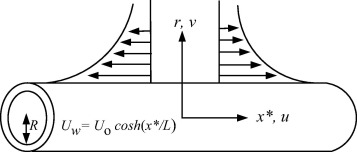Figure 1. Geometry of the flow.

If we consider ${\textstyle x=0}$ and ${\textstyle K=0{\mbox{,}}}$ then Eq. (9) reduces to the Sakiadis flow equation 

 $f_{\eta \eta \eta }+{\frac {1}{2}}{ff}_{\eta \eta }=0{\mbox{,}}$
(12)

with boundary conditions

 $f(0)=0{\mbox{,}}f_{\eta }(0)=1{\mbox{,}}f_{\eta }(\infty )=$$0$
(13)

The quantities of physical significance are the skin friction coefficient and Nusselt number which are defined as

 $C_{f}={\frac {{\tau }_{w}}{\rho U_{w}^{2}}}{\mbox{,}}\quad Nu={\frac {{xq}_{w}}{k(T_{w}-T_{\infty })}}{\mbox{,}}$
(14)

where ${\textstyle {\tau }_{w}}$ be the wall shear stress and ${\textstyle q_{w}}$ the constant heat flux from the surface, which are defined as

 ${\tau }_{w}=\mu {\left({\frac {\partial u}{\partial r}}\right)}_{r=R}{\mbox{,}}\quad q_{w}=$$-k{\left({\frac {\partial T}{\partial r}}\right)}_{r=R}{\mbox{.}}$
(15)

After using Eq. (15) into Eq. (14), the local skin friction coefficient and local Nusselt number take the form

 $C_{f}\quad {Re}_{x}^{1/2}=f_{\eta \eta }(0{\mbox{,}}x){\mbox{,}}\quad Nu\quad {Re}_{x}^{-1/2}=$$-{\theta }_{\eta }(0{\mbox{,}}x){\mbox{.}}$
(16)

## 3. Solution methodology

To solve nonlinear system of partial differential Eqs. (9) and (10) subject to the set of boundary conditions (11), we employed a very accurate and efficient implicit finite difference method commonly known as Keller box which is explained in detail in the book of Cebeci and Bradshaw . In present problem, the solution is obtained through following steps.

• Step-1: Eqs. (9) and (10) are written in terms of first order differential equations. Then functions and derivatives are replaced by central difference form in both coordinate directions. This results in a set of nonlinear difference equations for the number of unknown equal to the number of difference equations.
• Step-2: obtained nonlinear difference equations are then linearized by using Newton’s quasi-linearization technique.
• Step-3: and are solved using block tri-diagonal algorithm.

Now to initiate the process at ${\textstyle x=0}$, it is first provided guess profile for all five dependent variables arising after reduction to first order system and then obtained the solution outlined in steps 1-3. Iteration process is continued for all values of ${\textstyle x}$, until the difference in velocity and temperature profiles between the consecutive iteration is less than ${\textstyle {10}^{-5}}$. The accuracy of the employed numerical scheme has been established in Table 1 by comparison with the known results obtained by Rees and Pop  for flat plate ${\textstyle (x=0){\mbox{.}}}$ This comparison gives us confidence that the developed code is correct and has achieved the desired level of accuracy. Moreover, the values of ${\textstyle C_{f}\quad {Re}_{x}^{1/2}}$ and ${\textstyle Nu\quad {Re}_{x}^{-1/2}}$ are given in Table 2.

Table 1. Comparison with Rees and Pop .
${\textstyle x=0}$ ${\textstyle C_{f}\quad {Re}_{x}^{1/2}}$ ${\textstyle Nu\quad {Re}_{x}^{-1/2}}$
Ref.  −0.4439 −0.3509
Present −0.4439 −0.3509

Table 2. Numerical values of ${\textstyle C_{f}\quad {Re}_{x}^{1/2}}$ and ${\textstyle Nu\quad {Re}_{x}^{-1/2}}$ for various values of ${\textstyle K}$ when ${\textstyle Pr=0.7}$ at x = 0.5.
${\textstyle K}$ ${\textstyle C_{f}{Re}_{x}^{1/2}}$ ${\textstyle Nu\quad {Re}_{x}^{-1/2}}$
0 −0.55387 0.41012
0.5 −0.71911 0.60043
1 −0.88994 0.78894
1.5 −1.05690 0.96676

## 4. Result and discussion

An inspection of the governing partial differential equations indicates the presence of two emerging parameters: (a) the curvature parameter ${\textstyle K}$, (b) and the Prandtl number Pr.

The effects of these parameters are discussed in the forthcoming figures. In Figure 2 and Figure 3, effects of curvature parameter ${\textstyle K}$ on velocity and temperature profile are plotted. It is observed that curvature has significant effects on velocity and temperature profiles. In Fig. 2 the trend for velocity profile is rapidly decreasing in the region (${\textstyle 0<\eta <0.42}$) and then increasing after ${\textstyle \eta =0.42}$ for increasing values of curvature parameter ${\textstyle K}$ and consequently as a result the boundary layer thickness increases with the increase in curvature of the cylinder. This behavior of the fluid velocity is due to the fact that curvature ${\textstyle K}$ and radius of cylinder have reciprocal relationship. In other words increase in ${\textstyle K}$ tends to decrease in radius of cylinder. In this way, due to lesser surface area of the cylinder, the increase in velocity gradient at the surface increases and consequently enhances the shear stress per unit area. Fig. 2 also depicts that an increase in the curvature of the cylinder leads to augment in boundary layer thickness, as compared to the flat plate case (${\textstyle K=0}$). In Fig. 3 the temperature profiles decrease near the surface of the cylinder as ${\textstyle K}$ increases, and afterward rise significantly and the thermal boundary-layer thickness increases. This variation of the temperature profile is due to the reason that as the curvature increases, the radius of cylinder reduces the surface area which is intact with the fluid also decreases. It is also important to mention that heat is transferred into the fluid in modes: conduction at the cylinder surface and convection for the region ${\textstyle \eta >0}$. Now, as the area of surface of the cylinder decreases, a slender reduction in the temperature profile occurs close to the surface of the cylinder, since a smaller amount of heat energy is transferred from the surface to the fluid through conduction phenomena. On the other hand, the thermal boundary-layer thickness increases, because of the heat transport in the fluid due to enhanced convection process all around the cylinder, which is evident from Fig. 3. Fig. 4 is plotted to observe the consequence of curvature parameter ${\textstyle K}$ on the coefficient of skin friction ${\textstyle C_{f}\quad {Re}_{x}^{1/2}}$. It is noticed that as ${\textstyle K}$ increases, the wall skin friction coefficient ${\textstyle C_{f}\quad {Re}_{x}^{1/2}}$ decreases. This is due to the reason that, for larger curvature of the cylinder, the velocity gradient at the surface becomes increased rapidly as compared to the flat plate (${\textstyle K=0}$). Fig. 5 is plotted to observe the consequence of curvature parameter ${\textstyle K}$ on the Nusselt number ${\textstyle Nu\quad {Re}_{x}^{-1/2}}$. It is noticed that as ${\textstyle K}$ increases the Nusselt number ${\textstyle Nu\quad {Re}_{x}^{-1/2}}$ also increases. In Figure 6 and Figure 7, it is observed that both velocity and temperature boundary layer thickness decrease by increasing the value of ${\textstyle x}$. The effects of different values of Prandtl number Pr on temperature profile have been discussed in Fig. 8. It is observed that temperature is rapidly decreasing for increasing Pr and thermal boundary layer thickness decreases. It is obvious from the graph that fluids with low Prandtl number slow down the cooling process and fluids with high Prandtl number speed up the cooling process. The behavior of temperature profile shows that Prandtl number can be used to control the cooling process. This could be managed by using high Prandtl number fluids such as oils and lubricants. Fig. 9 shows that Nusselt number ${\textstyle Nu\quad {Re}_{x}^{-1/2}}$ increases along the surface of cylinder and with the increase of Pr. This is because of fact that by increasing the value of Pr thermal boundary layer decreases and heat transfer rate enhances. This finding also testify the results shown in Fig. 8.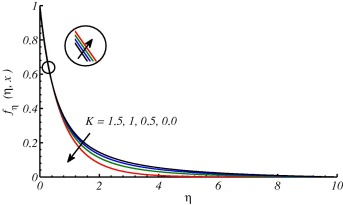Figure 2. Effects on velocity profile due to different values of ${\textstyle K}$ at ${\textstyle x=3}$.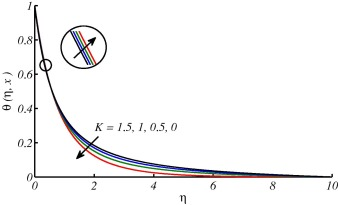Figure 3. Effects on temperature profile due to different values of ${\textstyle K}$ while ${\textstyle Pr=0.7}$ and ${\textstyle x=3}$.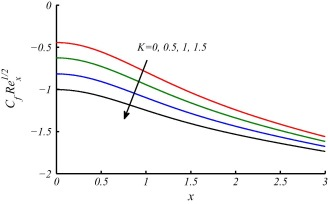Figure 4. Variations in Skin-friction coefficient for different values of ${\textstyle K}$ against ${\textstyle x}$.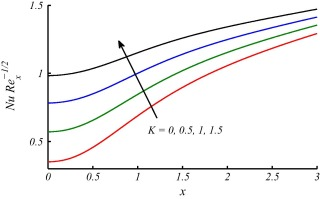Figure 5. Variations in Nusselt number for different values of ${\textstyle K}$ against ${\textstyle x}$ while ${\textstyle Pr=0.7}$.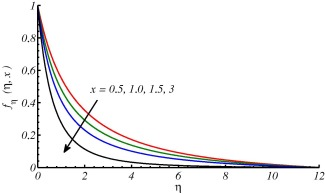Figure 6. Velocity profile for different values of ${\textstyle x}$ at ${\textstyle K=0.5}$.Figure 7. Temperature profile for different values of ${\textstyle x}$ at ${\textstyle K=0.5}$ and ${\textstyle Pr=0.7}$.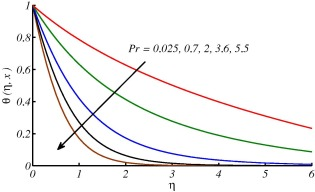Figure 8. Temperature profile for different value of ${\textstyle Pr}$ at ${\textstyle x=3}$.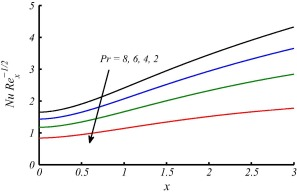Figure 9. Variation in Nusselt number for different values of ${\textstyle Pr}$ against ${\textstyle x}$.

## 5. Entropy generation analysis

Using the boundary layer approximation, the local volumetric rate of entropy generation EG for a Newtonian fluid over a hyperbolic stretching cylinder is defined as follows:

 $E_{G}={\underset {\mbox{Entropy effects due to heat transfer}}{\underbrace {{\frac {k}{T_{\infty }^{2}}}{\left({\frac {\partial T}{\partial r}}\right)}^{2}} }}+$${\underset {\mbox{Entropy effects due to fluid friction}}{\underbrace {{\frac {\mu }{T_{\infty }}}{\left({\frac {\partial u}{\partial r}}\right)}^{2}} }}$
(17)

After using results of Eq. (5) and Eq. (6) in Eq. (17), following form is obtained

 $E_{G}={\frac {{kr}^{2}{\left(T_{w}-T_{\infty }\right)}^{2}U_{0}coshx{\left({\theta }_{\eta }(\eta {\mbox{,}}x)\right)}^{2}}{{LR}^{2}T_{\infty }^{2}x\nu }}+$${\frac {r^{2}\mu {\left(U_{0}coshx\right)}^{3}{\left(f_{\eta \eta }(\eta {\mbox{,}}x)\right)}^{2}}{{LR}^{2}T_{\infty }x\nu }}$
(18)

Above equation can be written as

 $N_{E}^{_{\ast }}={\frac {E_{G}}{E_{0}}}={\frac {(1+2K\eta ){Re}_{L}coshx}{x}}\left[{\left({\theta }_{\eta }(\eta {\mbox{,}}x)\right)}^{2}+\right.$$\left.{\frac {Br{\left(coshx\right)}^{2}{\left(f_{\eta \eta }(\eta {\mbox{,}}x)\right)}^{2}}{{\Omega }^{_{\ast }}}}\right]$
(19)

where ${\textstyle N_{E}^{_{\ast }}}$ is the entropy generation number which is the ratio of local volumetric rate of entropy generation ${\textstyle E_{G}}$ and characteristic entropy generation rate ${\textstyle E_{0}=k{\left(T_{w}-T_{\infty }\right)}^{2}/L^{2}T_{\infty }^{2}}$, ${\textstyle {Re}_{L}=U_{0}L/\nu }$ is the Reynolds number, ${\textstyle Br=\mu U_{0}^{2}/k(T_{w}-T_{\infty })}$ is the Brinkman number, and ${\textstyle {\Omega }^{_{\ast }}=(T_{w}-T_{\infty })/T_{\infty }}$ is the dimensionless temperature difference and the ratio ${\textstyle Br{\Omega }^{{_{\ast }}-1}}$ is group parameter. The Bejan number Be serves as a substitute of entropy generation parameter and it represents the ratio between the entropy generation due to heat transfer and the total entropy generation due to heat transfer and fluid friction. It is defined by

 $Be={\frac {N_{EHT}}{N_{EHT}+N_{EFF}}}$
(20)

Here

 $N_{EHT}=(1+2K\eta ){Re}_{L}{\frac {coshx}{x}}{\left({\theta }_{\eta }(\eta {\mbox{,}}x)\right)}^{2}$
(21)
 $N_{EFF}=(1+2K\eta ){Re}_{L}{\frac {{cosh}^{3}x}{x}}\quad Br{\Omega }^{{_{\ast }}-1}{\left(f_{\eta \eta }(\eta {\mbox{,}}x)\right)}^{2}$
(22)

Bejan number Be   can also be written as ${\textstyle Be=1/1+{\Phi }^{_{\ast }}}$, where ${\textstyle {\Phi }^{_{\ast }}=N_{EFF}/N_{EHT}}$ is called irreversibility ratio.

Figure 10, Figure 11, Figure 12, Figure 13, Figure 14, Figure 15 and Figure 16 are drawn to discuss the influence of Prandtl number ${\textstyle Pr}$, curvature parameter ${\textstyle K}$, group parameter ${\textstyle Br{\Omega }^{{_{\ast }}-1}}$ and Reynolds number ${\textstyle {Re}_{L}}$ on entropy generation number ${\textstyle N_{E}^{_{\ast }}}$ and Bejan number ${\textstyle Be}$. In Figure 10 and Figure 11, the overall effect of Prandtl number is the enhancement in ${\textstyle N_{E}^{_{\ast }}}$ and ${\textstyle Be}$. This is because the temperature gradient increases with the larger values of ${\textstyle Pr}$. It is also noted that when ${\textstyle Pr<1}$ a small variation in values of ${\textstyle Be}$ at surface of the cylinder is observed and it increases for larger ${\textstyle \eta }$ and attains maximum value i.e., ${\textstyle Be\rightarrow 1}$. On the other hand when ${\textstyle Pr>1}$, large values of ${\textstyle Be}$ at surface of the cylinder are reported. These effects start decreasing and ${\textstyle Be\rightarrow 0}$ far away from the surface. Figure 12 and Figure 13 are plotted to see the effects of ${\textstyle K}$ on ${\textstyle N_{E}^{_{\ast }}}$ and ${\textstyle Be}$. Both figures depict that entropy generation is more dominant for stretching cylinder case ${\textstyle K>0}$ in comparison with flat plat case ${\textstyle K=0}$. In Fig. 13, ${\textstyle Be}$ decrease at the surface stretching cylinder (${\textstyle \eta =0}$) and reverse behavior is observed far away from the surface. The variations of group parameter ${\textstyle Br{\Omega }^{{_{\ast }}-1}}$ on ${\textstyle N_{E}^{_{\ast }}}$ and ${\textstyle Be}$ are depicted in Figure 14 and Figure 15. The enhancement in ${\textstyle Br{\Omega }^{{_{\ast }}-1}}$ results in augmentation of ${\textstyle N_{E}^{_{\ast }}}$ and these effects are opposite on ${\textstyle Be}$. The values of ${\textstyle Be}$ are decreasing with increase in ${\textstyle Br{\Omega }^{{_{\ast }}-1}}$ because the fluid friction dominates when ${\textstyle Br{\Omega }^{{_{\ast }}-1}}$ increases and this results in decrement of ${\textstyle Be}$. The effects of ${\textstyle {Re}_{L}}$ on entropy generation number are expressed in Fig. 16. This figure reveals that the entropy generation number has gained an increasing trend with increase in ${\textstyle {Re}_{L}}$.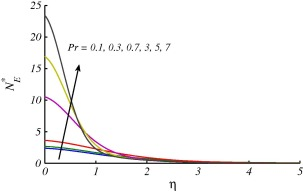Figure 10. Effects of ${\textstyle Pr}$ on ${\textstyle N_{E}^{_{\ast }}}$ when ${\textstyle K=0.5{\mbox{,}}\quad {Re}_{L}=2{\mbox{,}}\quad Br{\Omega }^{{_{\ast }}-1}=}$$1$ at ${\textstyle x=0.2}$.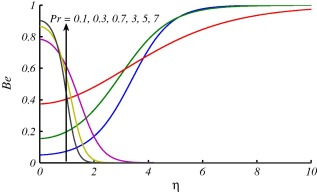Figure 11. Effects of ${\textstyle Pr}$ on ${\textstyle Be}$ when ${\textstyle K=0.5{\mbox{,}}\quad {Re}_{L}=2{\mbox{,}}\quad Br{\Omega }^{{_{\ast }}-1}=}$$1$ at ${\textstyle x=0.2}$.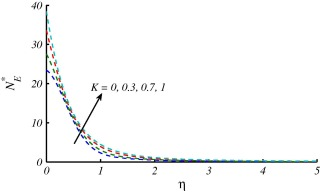Figure 12. Effects of ${\textstyle K}$ on ${\textstyle N_{E}^{_{\ast }}}$ when ${\textstyle {Re}_{L}=2{\mbox{,}}\quad Br{\Omega }^{{_{\ast }}-1}=1{\mbox{,}}\quad Pr=}$$7$ at ${\textstyle x=0.2}$.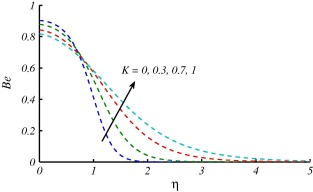Figure 13. Effects of ${\textstyle K}$ on ${\textstyle Be}$ when ${\textstyle Pr=7{\mbox{,}}\quad Br{\Omega }^{{_{\ast }}-1}=1}$ at ${\textstyle x=0.2}$.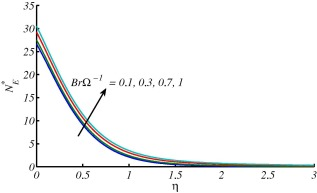Figure 14. Effects of ${\textstyle Br{\Omega }^{{_{\ast }}-1}}$ on ${\textstyle N_{E}^{_{\ast }}}$ when ${\textstyle Pr=7{\mbox{,}}\quad {Re}_{L}=2{\mbox{,}}\quad K=0.5}$ at ${\textstyle x=0.2}$.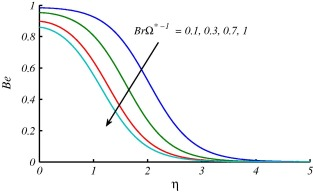Figure 15. Effects of ${\textstyle Br{\Omega }^{{_{\ast }}-1}}$ on ${\textstyle Be}$ when ${\textstyle K=0.5{\mbox{,}}\quad {Re}_{L}=2{\mbox{,}}\quad Pr=7}$ at ${\textstyle x=0.2}$.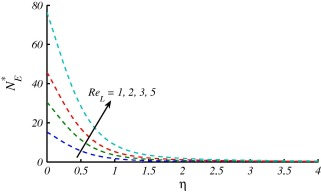Figure 16. Effects of ${\textstyle {Re}_{L}}$ on ${\textstyle N_{E}^{_{\ast }}}$ when ${\textstyle K=0.5{\mbox{,}}\quad Pr=7{\mbox{,}}\quad Br{\Omega }^{{_{\ast }}-1}=}$$1$ at ${\textstyle x=0.2}$.

## 6. Concluding remarks

A study is performed to investigate the flow and heat transfer characteristics over a nonlinear stretching cylinder. The nonlinear stretching velocity is considered as hyperbolic function. An analysis of entropy generation is also presented and results are computed numerically with Keller box method. From above study it is observed that:

• Increase in the values of curvature parameter ${\textstyle K}$ leads to increase in velocity and temperature distribution.
• skin-friction coefficient reduces and Nusselt number enhances with an increase in curvature parameter ${\textstyle K}$.
• The temperature reduces and Nusselt number enhances by increasing Prandtl number ${\textstyle Pr}$.
• Increasing trend of curvature parameter, Prandtl number and group parameter results in enhancement of entropy generation.

## Acknowledgment

The authors are grateful to reviewers for their constructive guideline which definitely improve this version of manuscript.

### Document informationPublished on 12/04/17

Licence: Other

### Document Score0

Views 28
Recommendations 0